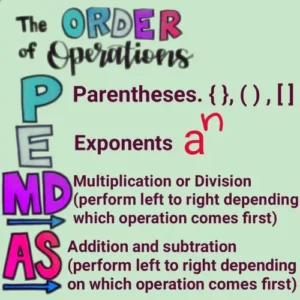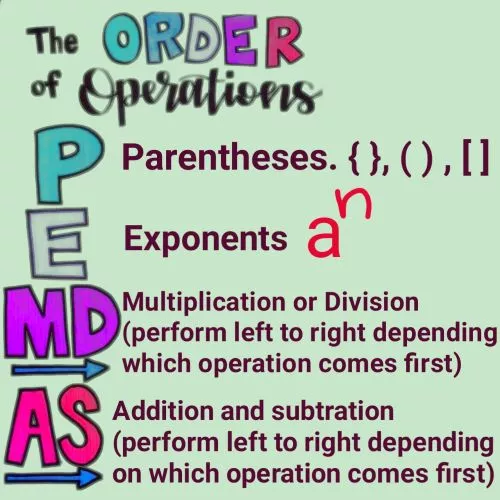December 8, 2023

# PEMDAS Examples: PEMDAS math rule, Definition , Examples and PEMDAS worksheet PDF Download

PEMDAS math rule, Definition and PEMDAS examples

Contents

# What is ‘PEMDAS’  ?PEMDAS  rule, Definition and  PEMDAS examples

‘PEMDAS’ is an acronym  for the order of operations.  Each letter represent an operation to calculate the value of mathematical expressions. The operations included in the PEMDAS  are performed from left to right. PEMDAS Stands for  P: Parentheses, E: Exponents ,  M:Multiplication , D: Division,  A: Addition and S: Subtraction

 P E M/D A/D Parentheses {} , [] ,  () Exponents multiplication /division (perform left to right depending on which operation comes first) addition/subtraction (perform left to right depending on which operation comes first)

The following rule must be followed if you want to use PEMDAS  correctly.

## PEMDAS RULE

(i) Perform operations inside of parentheses (brackets) before you do anything else.

(ii) After performing operations inside of parentheses , solve the exponents  (if there are any).

(iii) After the parentheses  and the exponents, perform multiplication or division from left to right depending on which operation comes first.

• Note: Just because M comes before D in the PEMDAS rule doesn’t mean that you will always perform multiplication before division.

(iv) Finally, after multiplication and division,  perform addition and subtraction from left to right depending on which operation comes first. The following acronym will help you remember the PEMDAS Rule.

The following examples show how to correctly apply  PEMDAS rule while solving mathematical operations.

### PEMDAS EXAMPLES WITH ANSWERS  (solved)

(i) 28 ÷

Sol.  28 ÷

=28 ÷                                            ( solving parentheses first :  9-7=2)

= 28 ÷4                                                 ( solving exponents

=4)

=7                                                           ( finally division)

(ii)  75-10 x 6

Sol. 75-10 x 6= 75- 60                    ( solving multiplication first  10 x 6=60)

= 15                             ( performing subtraction)

(iii)  8 x 8 ÷ 16

Sol. There are only two operations in the given mathematical expressions , multiplication  and division. According to PEMDAS ,  perform multiplication or division from left to right depending on which operation comes first. So in the given problem , we will solve multiplication  first then division as follows.

8 x 8 ÷ 16  = 64 ÷ 16

=4

(iv) 6 x 4 ÷ 12 +72÷ 8-9

Sol.  6 x 4 ÷ 12 +72÷ 8-9      =  24 ÷ 12 +72÷ 8-9                ( performing multiplication)

=  2 +9 -9                                        (performing division)

=2                                                     (performing subtraction)

(v) + (2 +12 x 2) -16 ÷ 4

Sol.

+ (2 +12 x 2) -16 ÷ 4  =  + 26-16 ÷ 4                 ( Solving operations inside parentheses)

=    9+ 26-16 ÷ 4                    (solving exponent

)

=9+ 26-4                                 ( performing division)

= 31

(vi)  8+14 ÷ 7 x 3-5

Sol. 8+14 ÷ 7 x 3-5  = 8+2  x 3-5                                        (performing division first)

= 8+6-5                                                 (performing multiplication)

= 14-5

=9

(vii)  12 – 8 + 4 + 9 × (40 ÷ 4)

Sol. 12 – 8 + 4 + 9 × (40 ÷ 4) = 12-8+4+9 x 10                       (solving division inside parentheses  40 ÷4=10)

= 12 – 8+4 +90                           (multiply  9 x 10 )

=  4+4+90                                      (subtracting 12-8 )

=98

(viii)  (42 ÷ 7 ) + 7 + ( 17 – 9 ) × 8

Sol. (42 ÷ 7 ) + 7 + ( 17 – 9 ) × 8    =  6 + 7 + ( 17 – 9 ) × 8                                     (Solving division  42 ÷ 7  )

=  6 + 7 + 8 × 8                                                     (performing subtraction   17-9  )

= 6+7 +64                                                                  (multiplying  8 x 8 )

= 77

(ix)  (10+43-5) ÷  6+

Sol.   (10+43-5) ÷  6+

=  48 ÷  6+                                               (Solving the expressions inside the parentheses)

=48 ÷  6+25                                                 (solving the exponent

)

= 8+25                                                            (performing 48 ÷  6)

= 33

(x) +(9-10÷ 5)

Sol.

+(9-10÷ 5)  =   +(9-10÷ 5)

=

+(9-2)

= + 7

= 81+7 = 88

(XI) 3 x 19 x 14  +18 ÷ 2

Sol.  3 x 19 x 14  +18 ÷ 2= 57  x 14  +18 ÷ 2

=798+18 ÷ 2

=798+9

= 807

(XII)  8 × 9 − 8 ÷ 2 + 14

Sol. 8 × 9 − 8 ÷ 2 + 14=  72 − 8 ÷ 2 + 14                         (performing multiplication)

=72 − 4+ 14                                      (dividing 8 ÷ 2 )

=68+14

=82

(XIII) 7 + 4 × 4 − 36 ÷ 9

Sol. 7 + 4 × 4 − 36 ÷ 9  = 7 + 16 − 36 ÷ 9                                  (performing multiplication)

= 7 + 16 − 4                                           (solving division)

=23-4

=19

(XIV)

Sol.                         (solving the subtraction lying inside the small brackets)

=

(solving exponent )

=

(performing multiplication inside the parentheses)

=[21-6] x 6

=15 x 6

=30

(XV)

Sol.

=62-  2 x 16 +1

=62- 32 +1

=30+1

=31

#### PEMDAS   EXAMPLES  WITH ANSWERS  (Unsolved)

(i) 10 ÷  (3+2) x 4 + +6-9                                             Ans.   30

(ii) 25-

+3 x 4                                                                Ans.    21

(iii)  3+ [6(11+1-4)]÷ 8 x 2                                             Ans.   15

(iv) 3 x (3+7) – ÷2                                                        Ans.   22

(v) 2 x

÷(26-1)                                                   Ans.  33

(vi)  (4+3) x 10 ÷  2 +(5+6)                                            Ans.   46

(vii)                                                            Ans.  142

(viii)

Ans.   148

(ix) (10-7) + (2 x 14 ÷ 4)                                             Ans.   10

(x) (10+2) ÷ 4 x [(15÷ 3)-4]                                      Ans.     3

(xi) 4(15÷ 3) +(6 x 3) –                                           Ans.    34

(xii) 4 × 6 ÷ 2 −

+ 15                                              Ans.   -37

(xiii) 8 − + 4 × 64 ÷

Ans .   20

(xiv)                                              Ans.    95

(xv)

(22 ÷11)  –               Ans.    -60

##### PEMDAS examples worksheet with answers PDF

###### PEMDAS QUIZ

2
Created onPEMDAS QUIZ

1 / 10

1.   14+18 ÷2 x 18 -7

2 / 10

2.  15 x 18  +12 ÷ 3 +9

3 / 10

8 x 4 +9 -9+18

4 / 10

11 x 11 - 6 x 17 +4

5 / 10

5.  +(9-10 ÷ 5)

6 / 10

÷ (24-4)

7 / 10

2-20÷ 5 x 3

8 / 10

8.

9 / 10

(19-8) x (10+4) +

10 / 10

(10+43-5) ÷6 +